## Excel ROUNDDOWN Function

Excel CEILING Function
The Excel CEILING function returns a given number rounded up to the nearest multiple of a given number of significance. The syntax of the CEILING function is as below:= CEILING (number, significance)…This post will guide you how to use Excel ROUNDDOWN function with syntax and examples in Microsoft excel.

### Description

The Excel ROUNDDOWN function round the number down to the specified number of digits. So you can use this function to round a given number down towards zero to a specified number of decimal point.

The ROUNDDOWN function is a build-in function in Microsoft Excel and it is categorized as a Math and Trigonometry Function.

The ROUNDDOWN function is available in Excel 2016, Excel 2013, Excel 2010, Excel 2007, Excel 2003, Excel XP, Excel 2000, Excel 2011 for Mac.

### Syntax

The syntax of the ROUNDDOWN function is as below:

`=ROUNDDOWN (number, num_digits)`

Where the ROUNDDOWN function arguments are:

• number -This is a required argument. Any real number that you want to round down.
• num_digits – This is a required argument. The number of digits to which numbe you wnat to round down.

Note:

• If num_digits argument is greater than 0, then the number is rounded down to the specified number of digits. So it will round down to the right of the decimal point.
• If num_digits argument is equal to 0, then the number is rounded down to the nearest integer.
• If num_digits argument is less than 0, then the number is rounded down to the left of the decimal point.

### Excel ROUNDDOWN Function Examples

The below examples will show you how to use Excel ROUNDDOWN Function to round a given number down to a specified number of decimal point.

1# to round a number 4.3 down to zero decimal places, enter the following formula in Cell B1.

`=ROUNDDOWN(4.3,0)`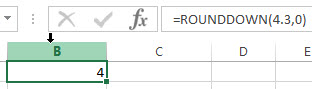2# to round a number 89.8 down to zero decimal places, enter the following formula in Cell B2.

`=ROUNDDOWN(89.8,0)`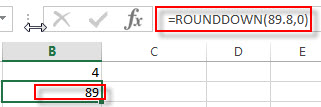3# to round a number 5.4329 down to two decimal places, enter the following formula in Cell B3.

`=ROUNDDOWN(5.432,2)`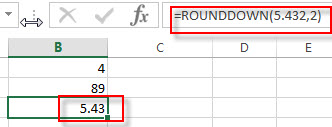4# to round a number 25.632 down to one decimal places to the left of the decimal point, enter the following formula in Cell B4.

`=ROUNDDOWN(25.632,-1)`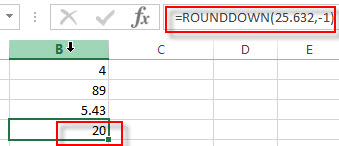### Related Functions

• Excel ROUND function
The Excel ROUND function rounds a number to a specified number of digits. You can use the ROUND function to round to the left or right of the decimal point in Excel.The syntax of the ROUND function is as below:=ROUND (number, num_digits)…
• Excel ROUNDUP function
The Excel ROUNDUP function rounds the number up to a specified number of decimal places. It will round away from 0.The syntax of the ROUNDUP function is as below:=ROUNDUP (number, num_digits)…
• Excel CEILING Function
The Excel CEILING function returns a given number rounded up to the nearest multiple of a given number of significance. The syntax of the CEILING function is as below:= CEILING (number, significance)…
• Excel CEILING.PRECISE Function
The Excel CEILING.PRECISE function returns a given number rounded up to the nearest multiple of a given number of significance or returns a number that is rounded up to the nearest integer number.The syntax of the CEILING.PRECISE function is as below:=CEILING.PRECISE (number, [significance])…

## How to Calculate Days, Weeks, Months and Years between Two Dates

This post will teach you how to calculate days, weeks, months and years between two dates in excel. How do I count the number of days, weeks, months and years between 2 dates in excel.

## Calculate days between two dates

If you want to calculate the difference in days between two dates, you can use the DATEDIF function to create an excel formula as follows:

`=DATEIF(B1,B2, "D")`

This formula will calculate days between two dates in cell B1 and B2, then returns the value in days.

## Calculate months between two dates

If you want to calculate the difference in months between tow dates, you can also use the DATEDIF function to create the following generic formula:

`=DATEDIF(B1,B2,"M")`

You should note that the third argument is “M” in the DATEDIF function. So this formula returns the value in months between two dates in excel.

## Calculate years between two dates

You can also use the DATEDIF function to calculate the number of years between two dates in excel, just refer to the following excel formula based on the DATEDIF function:

`=DATEDIF(B1,B2,"Y")`

This formula returns the value in years.

## Calculate weeks between two dates

If you want to calculate the number of weeks between two dates, you just need to subtract start date from the end date and then the returned result is divided by 7. So you can write the below generic formula:

`=(B2-B1)/7`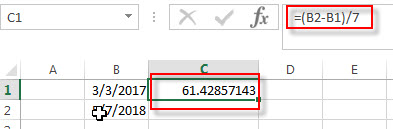This formula will return a decimal number, you can change the number format as you need.

Or you can use the INT function to rounds down to the nearest whole number or use ROUND function to round to nearest whole number.

=INT((B2-B1)/7)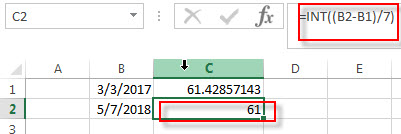=ROUND((B2-B1)/7,0)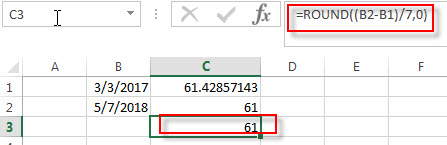You may be want to display the word “week” before the week number in the cell, you can do it as following steps:

1# right-click on the selected cells, then select Format Cells

2# on the Number Tab, choose Custom under Category, then select # “weeks” type.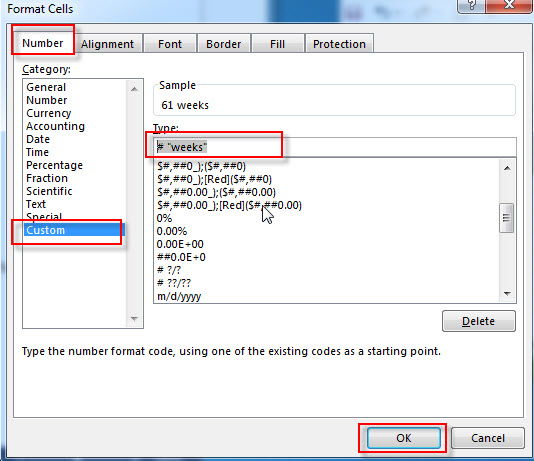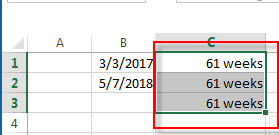## Calculate Years, Months and Days between two dates

If you want to determine how many years, months and days between two dates, you can use the DATEDIF function to create the following complex formula:

`=DATEDIF(B1,B2,"Y") & " Years, " & DATEDIF(B1,B2,"YM") & " Months, " & DATEDIF(B1,B2,"MD") & " Days"`

If you do not want to use the DATEDIF function, and you can also use the following formula to achieve the same result:

`=INT((TODAY()-A1)/365.25) & ” years , ” & INT(MOD((TODAY()-A1)/365.25,1)*12) & ” months and ” & INT(MOD((TODAY()-A1)/30.4375,1)*30.4375) & ” days”`

### Related Functions

• Excel INT function
The Excel INT function returns the integer portion of a given number. And it will rounds a given number down to the nearest integer.The syntax of the INT function is as below:= INT (number)…
• Excel DATEDIF function
The Excel DATEDIF function returns the number of days, months, or years between tow dates.The syntax of the DATEDIF function is as below:=DATEDIF (start_date,end_date,unit)…
• Excel TODAY function
The Excel TODAY function returns the serial number of the current date. So you can get the current system date from the TODAY function. The syntax of the TODAY function is as below:=TODAY()…
• Excel Round function
The Excel INT function rounds a number to a specified number of digits. You can use the ROUND function to round to the left or right of the decimal point in Excel.The syntax of the ROUND function is as below:=ROUND (number, num_digits)…

## How to calculate age from date of birth

This post explains that how to get age from the date of birth in a Cell. How to calculate birthdate to age using formulas in Excel. How to convert the birth of date to age quickly in Excel.

## Calculate age from date of birth

If you have a table that contains birthdate data in a range of Cells in Excel, and you want to get the age from those people’s birthdate value, you can use a combination of the INT function, the TODAY function to create an excel formula .

You can use the TODAY function to get the current date and then is subtracted to the value of birthdate and then the number is divided by 365 to get the exact age value, last, using the INT function to convert the age value to the integer.

Assuming that you want to calculate date of birth to age in Cell B2, you can use the following formula:

`=INT((TODAY()-B2)/365)`

Let’s see how this formula works:

=TODAY()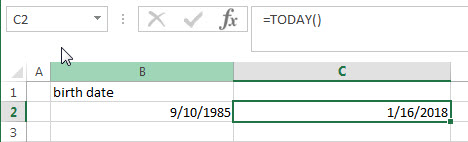This formula returns the current date.

=(TODAY()-B2)/365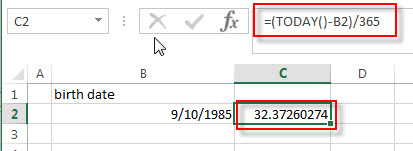The current date subtracts the date of birth to get the number of days of birth.  The returned number is divided by 365 (one year) to get the exact numbers of years.  Then it goes into the INT function as its argument.

=INT((TODAY()-B2)/365)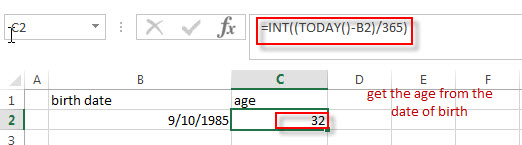This formula will round a decimal down to an integer. So you can get the age from the date of birth.

## Calculate age from date of birth with DATEDIF function

You can also create a formula based on the DATEIF function and TODAY function or NOW function. The DATEDIF function can return the number of complete year between the current date and birth date. So you can write down the following formula to convert date of birth to age quickly:

`=DATEDIF(B2,TODAY(),"Y")`

OR

`=DATEDIF(B2,NOW(),"Y")`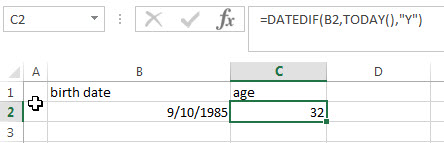## Calculate age from date of birth with YEARFRAC function

You can also create an excel formula based on the INT function or ROUNDDOWN function, the YEARFRAC function and the TODAY function to calculate age from birth of date.

The YEARFRAC function can be used to calculate the fraction of the year represented by the number of whole days between the date of birth and the current date.  Then you need to use the INT function or ROUNDDOWN function to round down the number returned by the YEARFRAC function to the integer value.

So you can write down the following formula:

`=INT(YEARFRAC(B2, TODAY(), 1))`

OR

`=ROUNDDOWN(YEARFRAC(B2, TODAY(), 1),0)`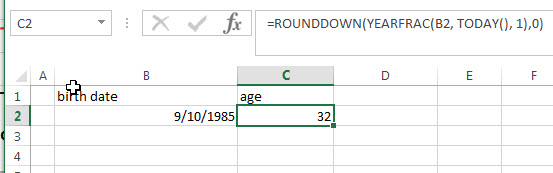### Related Formulas

• Convert Date to Day of Week in Excel
If you want to get the day name of the week as an abbreviation from a date, such as: Mon, Tue, etc. Enter the following TEXT formula in the Cell C1 that you want to get the day name of the week…
• Convet Text Date dd/mm/yy to mm/dd/yyyy as Date Format
If you want to convert dates in the text format to the date format as mm/dd/yyyy, you can use a combination of the DATE function, the VALUE function, the RIGHT function, the MID function, and the LEFT function to create a new excel formula….
• Convert date to month name with Text Function
If you want to convert the date to a month name, you can use the TEXT function with a specified format code like “mmm”in excel.  You can try to run the following TEXT formula:=TEXT(B1,”mmm”)…
• Convert Date to text with Text Function in Excel
you can use TEXT function in excel to convert dates to text in a specific format code. For example, The TEXT function can use the following patterns, like as: “mm/dd/yyyy”, “yyyy/mm/dd”, etc…
• Convert date to month and year only in excel
If you want to convert the date to month and year only, you can use “yyyymm” format code within the TEXT function in excel, so you can write down the below TEXT formula:=TEXT(date,”yyyymm”)…,
• Convert date to month and day only in excel
If you want to convert the date (mm/dd/yyyy) to month and day only, you can use “mm dd” format code within the TEXT function in excel, so you can write down the below TEXT formula: =TEXT(date,”mm dd”)…

### Related Functions

• Excel DATEDIF function
The Excel DATEDIF function returns the number of days, months, or years between tow dates.The syntax of the DATEDIF function is as below:=DATEDIF (start_date,end_date,unit)…
• Excel INT function
The Excel INT function returns the integer portion of a given number. And it will rounds a given number down to the nearest integer.The syntax of the INT function is as below:= INT (number)…